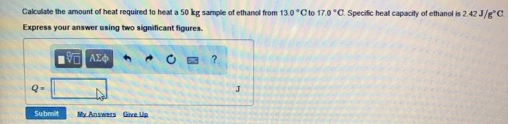Chemistry Calorimetry Solution: Calculate the amount of heat required to heat a 50...

# Solution: Calculate the amount of heat required to heat a 50 kg sample of ethanol from 13.0°C to 17.0°C. Specific heat capacity of ethanol is 2.42 J/g°C.

Problem

Calculate the amount of heat required to heat a 50 kg sample of ethanol from 13.0°C to 17.0°C. Specific heat capacity of ethanol is 2.42 J/g°C.View Complete Written Solution